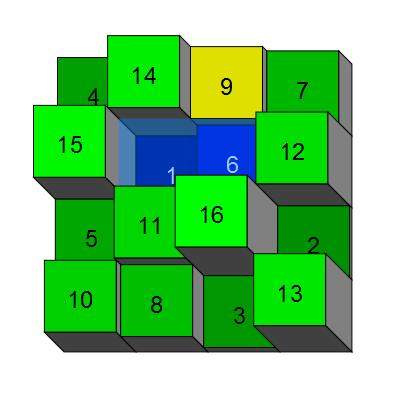# Is it a 4x4 Magic Square?

Logic Level 5Find the sum of all possible integral values of $n$ where $n \geq 2$, such that the numbers $1$ to $16$ can be written each in one square of a squared $4 \times 4$ paper (no repetitions allowed), such that each of the $8$ sums of the numbers in rows and columns is a multiple of $n$, and all of these $8$ multiples of $n$ are different from one other?

×

Problem Loading...

Note Loading...

Set Loading...